A_Bo 不怕别人比你聪明，就怕别人比你聪明还比你努力 2019-02-25T03:30:34.813Z http://yoursite.com/ A_Bo Hexo PAT_1091 http://yoursite.com//PAT/cjsjsbf890000csv91ik8xyp4/ 2019-02-25T03:26:01.000Z 2019-02-25T03:30:34.813Z 1091 Acute Stroke

题目描述

One important factor to identify acute stroke (急性脑卒中) is the volume of the stroke core. Given the results of image analysis in which the core regions are identified in each MRI slice, your job is to calculate the volume of the stroke core.

Input Specification:

Each input file contains one test case. For each case, the first line contains 4 positive integers: M, N, L and T, where M and N are the sizes of each slice (i.e. pixels of a slice are in an M×N matrix, and the maximum resolution is 1286 by 128); L (≤60) is the number of slices of a brain; and T is the integer threshold (i.e. if the volume of a connected core is less than T, then that core must not be counted).

Then L slices are given. Each slice is represented by an M×N matrix of 0’s and 1’s, where 1 represents a pixel of stroke, and 0 means normal. Since the thickness of a slice is a constant, we only have to count the number of 1’s to obtain the volume. However, there might be several separated core regions in a brain, and only those with their volumes no less than T are counted. Two pixels are connectedand hence belong to the same region if they share a common side, as shown by Figure 1 where all the 6 red pixels are connected to the blue one.

Output Specification:

For each case, output in a line the total volume of the stroke core.

思路

dfs程序只得到了25分，并没有满分，如果有修改并可以通过的地方，感觉大家的指点

dfs方法(25分)

]]>
<h1 id="1091-Acute-Stroke"><a href="#1091-Acute-Stroke" class="headerlink" title="1091 Acute Stroke"></a><a href="https://pintia.cn/problem-
PAT_1110 http://yoursite.com//PAT/cjsjsbf8o0005csv9qbba8753/ 2019-02-05T13:31:44.000Z 2019-02-05T13:49:39.716Z 1110 Complete Binary Tree

题目描述

Given a tree, you are supposed to tell if it is a complete binary tree.

Input Specification:

Each input file contains one test case. For each case, the first line gives a positive integer N (≤20) which is the total number of nodes in the tree – and hence the nodes are numbered from 0 to N−1. Then N lines follow, each corresponds to a node, and gives the indices of the left and right children of the node. If the child does not exist, a - will be put at the position. Any pair of children are separated by a space.

Output Specification:

For each case, print in one line YES and the index of the last node if the tree is a complete binary tree, or NO and the index of the root if not. There must be exactly one space separating the word and the number.

思路

思路一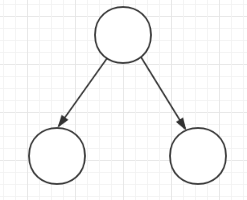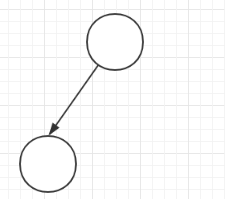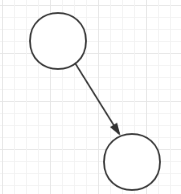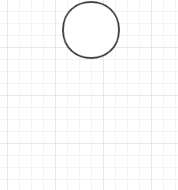参考博客

]]>
<h1 id="1110-Complete-Binary-Tree"><a href="#1110-Complete-Binary-Tree" class="headerlink" title="1110 Complete Binary Tree"></a><a href="ht
PAT_1109 http://yoursite.com//PAT/cjsjsbfgm000zcsv9mdwrj5r2/ 2019-02-04T03:42:39.000Z 2019-02-04T03:45:19.625Z 1109 Group Photo

题目描述

Formation is very important when taking a group photo. Given the rules of forming K rows with N people as the following:

• The number of people in each row must be N/K (round down to the nearest integer), with all the extra people (if any) standing in the last row;
• All the people in the rear row must be no shorter than anyone standing in the front rows;
• In each row, the tallest one stands at the central position (which is defined to be the position (m/2+1), where m is the total number of people in that row, and the division result must be rounded down to the nearest integer);
• In each row, other people must enter the row in non-increasing order of their heights, alternately taking their positions first to the right and then to the left of the tallest one (For example, given five people with their heights 190, 188, 186, 175, and 170, the final formation would be 175, 188, 190, 186, and 170. Here we assume that you are facing the group so your left-hand side is the right-hand side of the one at the central position.);
• When there are many people having the same height, they must be ordered in alphabetical (increasing) order of their names, and it is guaranteed that there is no duplication of names.

Now given the information of a group of people, you are supposed to write a program to output their formation.

Input Specification:

Each input file contains one test case. For each test case, the first line contains two positive integers N (≤104), the total number of people, and K (≤10), the total number of rows. Then N lines follow, each gives the name of a person (no more than 8 English letters without space) and his/her height (an integer in [30, 300]).

Output Specification:

For each case, print the formation – that is, print the names of people in K lines. The names must be separated by exactly one space, but there must be no extra space at the end of each line. Note: since you are facing the group, people in the rear rows must be printed above the people in the front rows.

程序

]]>
<h1 id="1109-Group-Photo"><a href="#1109-Group-Photo" class="headerlink" title="1109 Group Photo"></a><a href="https://pintia.cn/problem-set
PAT—_1137 http://yoursite.com//PAT/cjsjsbfh2001ccsv9jmntqchd/ 2019-02-02T10:14:31.000Z 2019-02-02T10:24:14.835Z 1137 Final Grading

题目描述

For a student taking the online course “Data Structures” on China University MOOC (http://www.icourse163.org/), to be qualified for a certificate, he/she must first obtain no less than 200 points from the online programming assignments, and then receive a final grade no less than 60 out of 100. The final grade is calculated by G=(Gmid−term×40%+Gfinal×60%) if Gmid−term>Gfinal, or Gfinal will be taken as the final grade G. Here Gmid−term and Gfinal are the student’s scores of the mid-term and the final exams, respectively.

The problem is that different exams have different grading sheets. Your job is to write a program to merge all the grading sheets into one.

Input Specification:

Each input file contains one test case. For each case, the first line gives three positive integers: P , the number of students having done the online programming assignments; M, the number of students on the mid-term list; and N, the number of students on the final exam list. All the numbers are no more than 10,000.

Then three blocks follow. The first block contains P online programming scores Gp’s; the second one contains M mid-term scores Gmid−term’s; and the last one contains N final exam scores Gfinal’s. Each score occupies a line with the format: StudentID Score, where StudentID is a string of no more than 20 English letters and digits, and Score is a nonnegative integer (the maximum score of the online programming is 900, and that of the mid-term and final exams is 100).

Output Specification:

For each case, print the list of students who are qualified for certificates. Each student occupies a line with the format:

StudentID Gp Gmid−term Gfinal G

If some score does not exist, output “−1” instead. The output must be sorted in descending order of their final grades (G must be rounded up to an integer). If there is a tie, output in ascending order of their StudentID‘s. It is guaranteed that the StudentID‘s are all distinct, and there is at least one qullified student.

程序

]]>
PAT_1133 http://yoursite.com//PAT/cjsjsbfm40027csv9vkm8eb5n/ 2019-02-02T04:42:24.000Z 2019-02-02T04:45:13.605Z 1133 Splitting A Linked List

题目描述

Given a singly linked list, you are supposed to rearrange its elements so that all the negative values appear before all of the non-negatives, and all the values in [0, K] appear before all those greater than K. The order of the elements inside each class must not be changed. For example, given the list being 18→7→-4→0→5→-6→10→11→-2 and K being 10, you must output -4→-6→-2→7→0→5→10→18→11.

Input Specification:

Each input file contains one test case. For each case, the first line contains the address of the first node, a positive N (≤105) which is the total number of nodes, and a positive K (≤103). The address of a node is a 5-digit nonnegative integer, and NULL is represented by −1.

Then N lines follow, each describes a node in the format:

where Address is the position of the node, Data is an integer in [−105,105], and Next is the position of the next node. It is guaranteed that the list is not empty.

Output Specification:

For each case, output in order (from beginning to the end of the list) the resulting linked list. Each node occupies a line, and is printed in the same format as in the input.

程序

]]>
PAT_1094 http://yoursite.com//PAT/cjsjsbf8q0006csv9t6hmdzkh/ 2019-02-01T12:46:59.000Z 2019-02-01T12:53:45.391Z 1094 The Largest Generation

题目描述

A family hierarchy is usually presented by a pedigree tree where all the nodes on the same level belong to the same generation. Your task is to find the generation with the largest population.

Input Specification:

Each input file contains one test case. Each case starts with two positive integers N (<100) which is the total number of family members in the tree (and hence assume that all the members are numbered from 01 to N), and M (<N) which is the number of family members who have children. Then M lines follow, each contains the information of a family member in the following format:

where ID is a two-digit number representing a family member, K (>0) is the number of his/her children, followed by a sequence of two-digit ID‘s of his/her children. For the sake of simplicity, let us fix the root ID to be 01. All the numbers in a line are separated by a space.

Output Specification:

For each test case, print in one line the largest population number and the level of the corresponding generation. It is assumed that such a generation is unique, and the root level is defined to be 1.

程序

]]>
<h1 id="1094-The-Largest-Generation"><a href="#1094-The-Largest-Generation" class="headerlink" title="1094 The Largest Generation"></a><a hr
PAT_1093 http://yoursite.com//PAT/cjsjsbf8m0004csv9zdxlib31/ 2019-02-01T10:17:48.000Z 2019-02-01T10:20:47.076Z 1093 Count PAT’s

题目描述

The string APPAPT contains two PAT‘s as substrings. The first one is formed by the 2nd, the 4th, and the 6th characters, and the second one is formed by the 3rd, the 4th, and the 6th characters.

Now given any string, you are supposed to tell the number of PAT‘s contained in the string.

Input Specification:

Each input file contains one test case. For each case, there is only one line giving a string of no more than 105 characters containing only P, A, or T.

Output Specification:

For each test case, print in one line the number of PAT‘s contained in the string. Since the result may be a huge number, you only have to output the result moded by 1000000007.

程序

]]>
<h1 id="1093-Count-PAT’s"><a href="#1093-Count-PAT’s" class="headerlink" title="1093 Count PAT’s"></a><a href="https://pintia.cn/problem-set
PAT_1146 http://yoursite.com//PAT/cjsjsbfh6001fcsv91wr5n87a/ 2019-02-01T08:33:04.000Z 2019-02-01T08:36:50.117Z 1146 Topological Order

题目描述

1146 Topological Order （25 分）

This is a problem given in the Graduate Entrance Exam in 2018: Which of the following is NOT a topological order obtained from the given directed graph? Now you are supposed to write a program to test each of the options.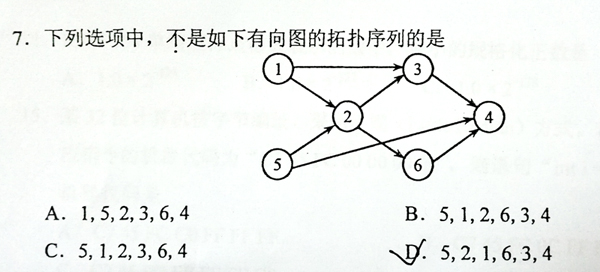Input Specification:

Each input file contains one test case. For each case, the first line gives two positive integers N (≤ 1,000), the number of vertices in the graph, and M (≤ 10,000), the number of directed edges. Then M lines follow, each gives the start and the end vertices of an edge. The vertices are numbered from 1 to N. After the graph, there is another positive integer K (≤ 100). Then K lines of query follow, each gives a permutation of all the vertices. All the numbers in a line are separated by a space.

Output Specification:

Print in a line all the indices of queries which correspond to “NOT a topological order”. The indices start from zero. All the numbers are separated by a space, and there must no extra space at the beginning or the end of the line. It is graranteed that there is at least one answer.

程序

]]>
<h1 id="1146-Topological-Order"><a href="#1146-Topological-Order" class="headerlink" title="1146 Topological Order"></a><a href="https://pin
PAT_1144 http://yoursite.com//PAT/cjsjsbfh8001icsv9ewk286ej/ 2019-02-01T06:36:46.000Z 2019-02-01T06:38:58.715Z 1144 The Missing Number

题目描述

1144 The Missing Number （20 分）

Given N integers, you are supposed to find the smallest positive integer that is NOT in the given list.

Input Specification:

Each input file contains one test case. For each case, the first line gives a positive integer N (≤105). Then N integers are given in the next line, separated by spaces. All the numbers are in the range of int.

Output Specification:

Print in a line the smallest positive integer that is missing from the input list.

程序

]]>
<h1 id="1144-The-Missing-Number"><a href="#1144-The-Missing-Number" class="headerlink" title="1144 The Missing Number "></a><a href="https:/
PAT_1118 http://yoursite.com//PAT/cjsjsbfh00018csv9428nhc5b/ 2019-01-30T06:21:24.000Z 2019-01-30T08:24:45.188Z 1118 Birds in Forest （25 分）

题目描述

Some scientists took pictures of thousands of birds in a forest. Assume that all the birds appear in the same picture belong to the same tree. You are supposed to help the scientists to count the maximum number of trees in the forest, and for any pair of birds, tell if they are on the same tree.

Input Specification:

Each input file contains one test case. For each case, the first line contains a positive number N (≤104) which is the number of pictures. Then N lines follow, each describes a picture in the format:

K B1 B2 … BK

where K is the number of birds in this picture, and Bi’s are the indices of birds. It is guaranteed that the birds in all the pictures are numbered continuously from 1 to some number that is no more than 104.

After the pictures there is a positive number Q (≤104) which is the number of queries. Then Q lines follow, each contains the indices of two birds.

Output Specification:

For each test case, first output in a line the maximum possible number of trees and the number of birds. Then for each query, print in a line Yes if the two birds belong to the same tree, or No if not.

参考博客

1118 Birds in Forest（25 分）

]]>
<h1 id="1118-Birds-in-Forest-（25-分）"><a href="#1118-Birds-in-Forest-（25-分）" class="headerlink" title="1118 Birds in Forest （25 分）"></a><a hr
PAT_1116 http://yoursite.com//PAT/cjsjsbfgy0016csv9qvbcp1kh/ 2019-01-29T14:35:16.000Z 2019-01-29T14:46:16.248Z 1116 Come on! Let’s C （20 分）

题目描述

“Let’s C” is a popular and fun programming contest hosted by the College of Computer Science and Technology, Zhejiang University. Since the idea of the contest is for fun, the award rules are funny as the following:

• 0、 The Champion will receive a “Mystery Award” (such as a BIG collection of students’ research papers…).
• 1、 Those who ranked as a prime number will receive the best award – the Minions (小黄人)!
• 2、 Everyone else will receive chocolates.

Given the final ranklist and a sequence of contestant ID’s, you are supposed to tell the corresponding awards.

Input Specification:

Each input file contains one test case. For each case, the first line gives a positive integer N (≤104), the total number of contestants. Then N lines of the ranklist follow, each in order gives a contestant’s ID (a 4-digit number). After the ranklist, there is a positive integer K followed by K query ID’s.

Output Specification:

For each query, print in a line ID: award where the award is Mystery Award, or Minion, or Chocolate. If the ID is not in the ranklist, print Are you kidding? instead. If the ID has been checked before, print ID: Checked.

写在后面

]]>
<h1 id="1116-Come-on-Let’s-C-（20-分）"><a href="#1116-Come-on-Let’s-C-（20-分）" class="headerlink" title="1116 Come on! Let’s C （20 分）"></a>1116
PAT_1122 http://yoursite.com//PAT/cjsjsbfgo0011csv9so0icul6/ 2019-01-29T10:21:19.000Z 2019-01-29T10:29:33.077Z 1122 Hamiltonian Cycle

The “Hamilton cycle problem” is to find a simple cycle that contains every vertex in a graph. Such a cycle is called a “Hamiltonian cycle”.

In this problem, you are supposed to tell if a given cycle is a Hamiltonian cycle.

Input Specification:

Each input file contains one test case. For each case, the first line contains 2 positive integers N (2<N≤200), the number of vertices, and M, the number of edges in an undirected graph. Then M lines follow, each describes an edge in the format Vertex1 Vertex2, where the vertices are numbered from 1 to N. The next line gives a positive integer K which is the number of queries, followed by K lines of queries, each in the format:

n V1 V2 … Vn

where n is the number of vertices in the list, and Vi’s are the vertices on a path.

Output Specification:

For each query, print in a line YES if the path does form a Hamiltonian cycle, or NO if not.

思路

1. 满足回路，即路径首尾相同
2. 经过所有节点且经过一次
3. 路径必须是连通的，即路径中相邻节点必须有路

参考资料

]]>
<h1 id="1122-Hamiltonian-Cycle"><a href="#1122-Hamiltonian-Cycle" class="headerlink" title="1122 Hamiltonian Cycle"></a>1122 Hamiltonian Cyc
PAT_1121 http://yoursite.com//PAT/cjsjsbfgt0014csv9skdmoviq/ 2019-01-29T07:49:42.000Z 2019-01-29T08:06:32.353Z 1121 Damn Single

题目描述

“Damn Single (单身狗)” is the Chinese nickname for someone who is being single. You are supposed to find those who are alone in a big party, so they can be taken care of.

Input Specification:

Each input file contains one test case. For each case, the first line gives a positive integer N (≤ 50,000), the total number of couples. Then N lines of the couples follow, each gives a couple of ID’s which are 5-digit numbers (i.e. from 00000 to 99999). After the list of couples, there is a positive integer M (≤ 10,000) followed by M ID’s of the party guests. The numbers are separated by spaces. It is guaranteed that nobody is having bigamous marriage (重婚) or dangling with more than one companion.

Output Specification:

First print in a line the total number of lonely guests. Then in the next line, print their ID’s in increasing order. The numbers must be separated by exactly 1 space, and there must be no extra space at the end of the line.

程序

]]>
<h1 id="1121-Damn-Single"><a href="#1121-Damn-Single" class="headerlink" title="1121 Damn Single"></a>1121 Damn Single</h1><h2 id="题目描述"><a
PAT_1090 http://yoursite.com//PAT/cjsjsbf8f0001csv9tbnuez3t/ 2019-01-29T03:13:00.000Z 2019-01-29T07:51:35.385Z 1090 Highest Price in Supply Chain

题目描述

A supply chain is a network of retailers（零售商）, distributors（经销商）, and suppliers（供应商）– everyone involved in moving a product from supplier to customer.

Starting from one root supplier, everyone on the chain buys products from one’s supplier in a price P and sell or distribute them in a price that is r% higher than P. It is assumed that each member in the supply chain has exactly one supplier except the root supplier, and there is no supply cycle.

Now given a supply chain, you are supposed to tell the highest price we can expect from some retailers.

Input Specification:

Each input file contains one test case. For each case, The first line contains three positive numbers: N (≤105), the total number of the members in the supply chain (and hence they are numbered from 0 to N−1); P, the price given by the root supplier; and r, the percentage rate of price increment for each distributor or retailer. Then the next line contains N numbers, each number Si is the index of the supplier for the i-th member. Sroot for the root supplier is defined to be −1. All the numbers in a line are separated by a space.

Output Specification:

For each test case, print in one line the highest price we can expect from some retailers, accurate up to 2 decimal places, and the number of retailers that sell at the highest price. There must be one space between the two numbers. It is guaranteed that the price will not exceed 1010.

程序

]]>
<h1 id="1090-Highest-Price-in-Supply-Chain"><a href="#1090-Highest-Price-in-Supply-Chain" class="headerlink" title="1090 Highest Price in Su